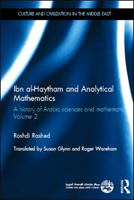# Sciences, Philosophie, Histoire – UMR 7219, laboratoire SPHERE

## Search

On this website

On the whole CNRS Web

Home > Publications > Grandes éditions > Textes mathématiques > Ibn al-Haytham and Analytical Mathematics

A History of Arabic Sciences and Mathematics, Volume 2, 2012

## Ibn al-Haytham and Analytical Mathematics

A History of Arabic Sciences and Mathematics, Volume 2, 2012This volume provides a unique primary source on the history and philosophy of mathematics and the exact sciences in the mediaeval Arab world. The second of five comprehensive volumes, this book offers a detailed exploration of Arabic mathematics in the eleventh century as embodied in the legacy of the celebrated polymath al-Hasan ibn al-Haytham.

Extensive analyses and annotations from the eminent scholar, Roshdi Rashed, support a number of key Arabic texts from Ibn al-Haytham’s treatises in infinitesimal mathematics, translated here into English for the first time. Rashed shows how Ibn al-Haytham’s works demonstrate a remarkable mathematical competence in mathematical subjects like the quadrature of the circle and of lunes, the calculation of the volumes of paraboloids, the problem of isoperimetric plane figures and solid figures with equal surface areas, along with the extraction of square and cubic roots.

The present text is complemented by the first volume of A History of Arabic Sciences and Mathematics, which focused on founding figures and commentators in the ninth and tenth centuries Archimedean-Apollonian mathematical ‘School of Baghdad’. This constellation of works illustrates the historical and epistemological development of ‘infinitesimal mathematics’ as it became clearly articulated in the oeuvre of Ibn al-Haytham.

Contributing to a more informed and balanced understanding of the internal currents of the history of mathematics and the exact sciences in Islam, and of its adaptive interpretation and assimilation in the European context, this fundamental text will appeal to historians of ideas, epistemologists and mathematicians at the most advanced levels of research.

:: Routledge, Series Culture and Civilization in the Middle East, 2012

:: London, Centre for Arab Unity Studies

:: 450 p.

:: ISBN 978-0-415-58218-6

Other volumes of the serie:

Contents

Preface, xi
Note, xiii
INTRODUCTION: IBN AL-HAYTHAM AND HIS WORK ON INFINITESIMAL MATHEMATICS

1. Ibn al-Haytham: from Basra to Cairo, 1

2. Al-Îasan ibn al-Îasan and MuÌammad ibn al-Îasan: mathematician and philosopher, 11

3. The works of al-Îasan ibn al-Haytham on infinitesimal mathematics, 25

CHAPTER I: THE QUADRATURE OF LUNES AND CIRCLES

• 1.1. INTRODUCTION, p. 39
• 1.2. MATHEMATICAL COMMENTARY, p. 42
• 1.2.1. Treatise on lunes, p. 42
• 1.2.2. Treatise on the quadrature of the circle, p. 46
• 1.2.3. Exhaustive treatise on the figures of lunes, p. 49
• 1.3. TRANSLATED TEXTS
• 1.3.1. Treatise on Lunes, p. 93
• 1.3.2. Treatise on the Quadrature of the Circle, p. 99
• 1.3.3. Exhaustive Treatise on the Figures of Lunes, p. 107

CHAPTER II: CALCULATION OF VOLUMES OF PARABOLOIDS AND SPHERES AND THE EXHAUSTION METHOD

• 2.1. INTRODUCTION, p. 143
• 2.2. MATHEMATICAL COMMENTARY, p. 144
• 2.2.1. Calculation of volumes of paraboloids, p. 144
• 2.2.2.1. Arithmetical lemmas, p. 144
• 2.2.2.2. Volume of a paraboloid of revolution, p. 151
• 2.2.2.3. The volume of the second species of paraboloid, p. 160
• 2.2.2.4. Study of surrounding solids, p. 164
• 2.2.3. Calculation of the volume of a sphere, p. 168
• 2.3. TRANSLATED TEXTS:
• 2.3.1. On the Measurement of the Paraboloid, p. 177
• 2.3.2. On the Measurement of the Sphere, p. 221
• 2.3.3. On the Division of Two Different Magnitudes as Mentioned
in the First Proposition of the Tenth Book of Euclid’s Elements
, p. 235

CHAPTER III: THE PROBLEMS OF ISOPERIMETRIC AND ISEPIPHANIC
FIGURES AND THE STUDY OF THE SOLID ANGLE

• 3.1. INTRODUCTION, p. 239
• 3.2. MATHEMATICAL COMMENTARY, p. 242

x CONTENTS
• 3.3. TRANSLATED TEXT: On the Sphere which is the Largest of all the Solid
Figures having Equal Perimeters and On the Circle which is the Largest
of all the Plane Figures having Equal Perimeters
, p. 305

APPENDIX: THE APPROXIMATION OF ROOTS

• 4.1. MATHEMATICAL COMMENTARY, p. 343
• 4.2. TRANSLATED TEXTS
• 4.3.1. On the Cause of the Square Root, its Doubling and its Displacement, p. 351
• 4.3.2. On the Extraction of the Side of a Cube, p. 357

SUPPLEMENTARY NOTES

• 1. On the Arithmetic of Transactions, p. 361
• 2. The Configuration of the Universe: a Book by al-Îasan ibn al-Haytham ? . 362
• 3. Ibn Sinæn and Ibn al-Haytham on the subject of ‘shadow lines’, p. 377
• 4. Commentary in the Resolution of Doubts by Ibn al-Haytham on
Proposition X.1 of the Elements, p. 381
• 5. List of Ibn al-Haytham’s works, p. 391

BIBLIOGRAPHY, p. 429

INDEXES

Index of names, p. 439

Subject index
Index of works